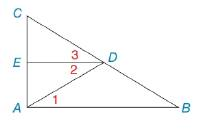Chapter 2.3, Problem 21EElementary Geometry For College St...

7th Edition
Alexander + 2 others
ISBN: 9781337614085

Solutions

Chapter
SectionElementary Geometry For College St...

7th Edition
Alexander + 2 others
ISBN: 9781337614085
Textbook Problem

In Exercise 19 to 22 complete the proof. Given: D E → bisects ∠ C D A ∠ 3 ≅ ∠ 1 Prove: E D ¯ ∥ A B ¯To determine

To find:

The complete proof for the given statement.

Explanation

Given:

The given statement is,

DE bisects CDA and 31.

Figure (1)

Theorem:

If two lines are cut by a transversal so that alternate interior angles are congruent, then these lines are parallel.

Approach:

The given statement is,

DE bisects CDA and 31.

Therefore,

23

Use Transitive Property of congruence.

21

1 and 2 are two congruent alternate interior angles.

Thus, ED¯AB¯.

The complete proof for the given statement is shown in the following table,

 Proof Statements Reasons 1. ∠2≅∠3 1. DE→ bisects ∠CDA 2. ∠3≅∠1 2

Still sussing out bartleby?

Check out a sample textbook solution.

See a sample solution

The Solution to Your Study Problems

Bartleby provides explanations to thousands of textbook problems written by our experts, many with advanced degrees!

Get Started

In Exercises 23-36, find the domain of the function. 24. f(x) = 7 x2

Applied Calculus for the Managerial, Life, and Social Sciences: A Brief Approach

Factoring Completely Factor the expression completely. 107. t2 6t + 9

Precalculus: Mathematics for Calculus (Standalone Book)

Find the derivative of the function. y=xxettdt

Single Variable Calculus: Early Transcendentals, Volume I

Evaluate limx0sin(3+x)2sin9x.

Calculus (MindTap Course List)

Differentiate the function. G(y)=ln(2y+1)5y2+1

Single Variable Calculus: Early Transcendentals

If , then h′(x) = 8t3 + 2t 24x2 + 2 8x3 + 2x – 3 8x3 + 2x

Study Guide for Stewart's Single Variable Calculus: Early Transcendentals, 8th

Explain the purpose of a research proposal and how it differs from a research report.

Research Methods for the Behavioral Sciences (MindTap Course List)